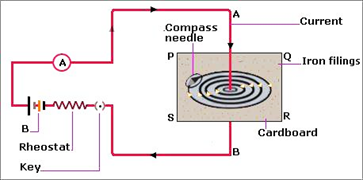### Question 12. Which of the statement given below correctly describes the magnetic field near a long, straight current-carrying conductor? a. The magnetic lines of force are in a plane, perpendicular to the conductor in the form of straight lines. b. The magnetic lines of force are parallel to the conductor on all the sides of the conductor.

Question 12.

Which of the statement given below correctly describes the magnetic field near a long, straight current-carrying conductor?

a. The magnetic lines of force are in a plane, perpendicular to the conductor in the form of straight lines.

b. The magnetic lines of force are parallel to the conductor on all the sides of the conductor.

c. The magnetic lines of force are perpendicular to the conductor going radially outward.

d. The magnetic lines of force are in concentric circles with the wire as the center, in a plane perpendicular to the conductor.